# NCERT Exemplar Solutions for Class 6 Maths Chapter 8 Ratio and Proportion

NCERT Exemplar Solutions for Class 6 Maths Chapter 8 Ratio and Proportion are provided here. NCERT Exemplar Solutions is the best material for students who face difficulties in solving problems. Our expert tutors formulate this exercise to assist you with your exam preparation, to attain good marks in Maths. We suggest students go through this NCERT Exemplar Solutions for Class 6 Maths and gain more knowledge.

Chapter 8 – Ratio and Proportion contain questions related to the ratio in simplest form and comparison of ratios. The ratio of two quantities of the same kind and in the same units is the fraction that one quantity is of others. The ratio ‘a’ is to ‘b’ is the fraction (a/b), written as a: b. Some of the important topics covered in this chapter are ratio, proportion, equivalent and unitary method.

## Download the PDF of NCERT Exemplar Solutions for Class 6 Maths Chapter 8 Ratio and Proportion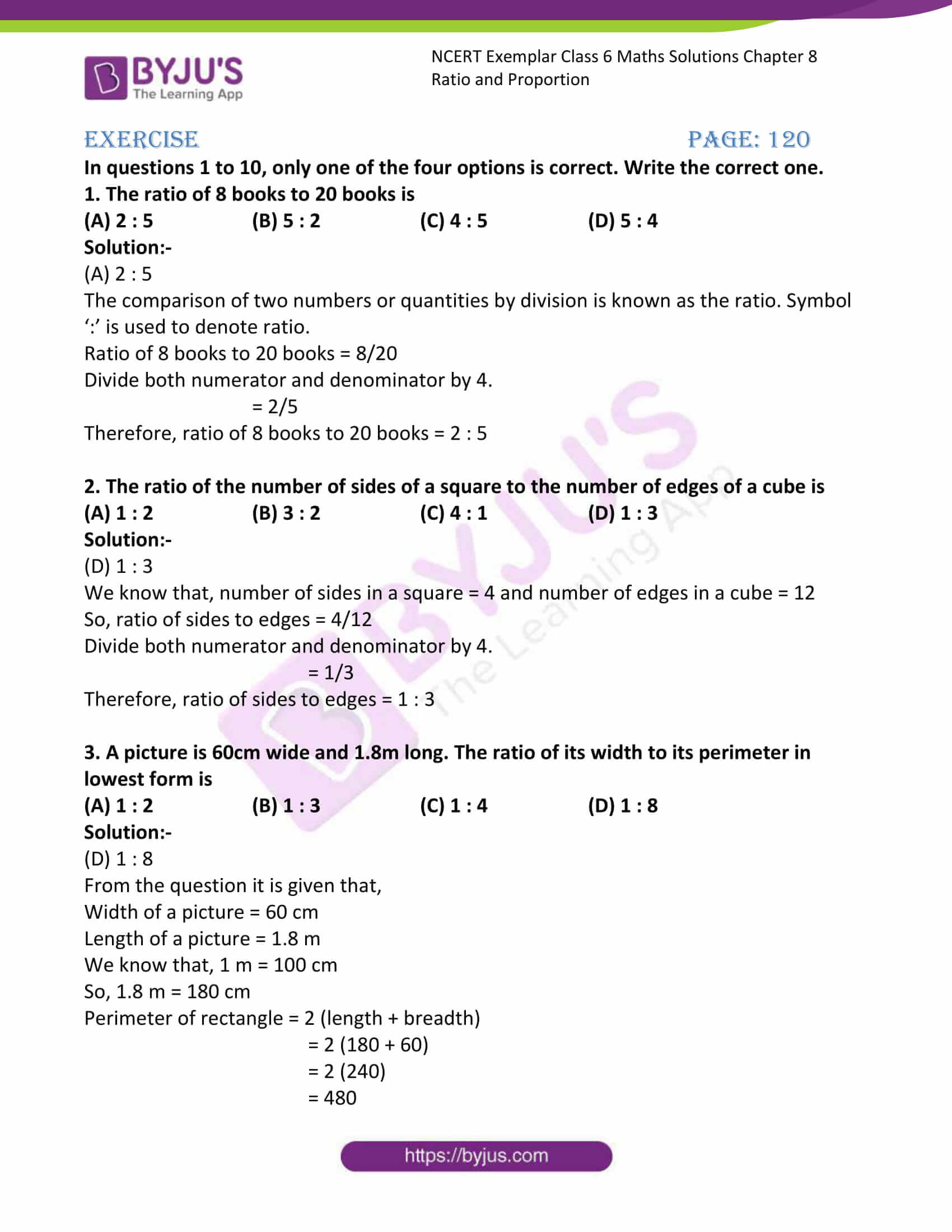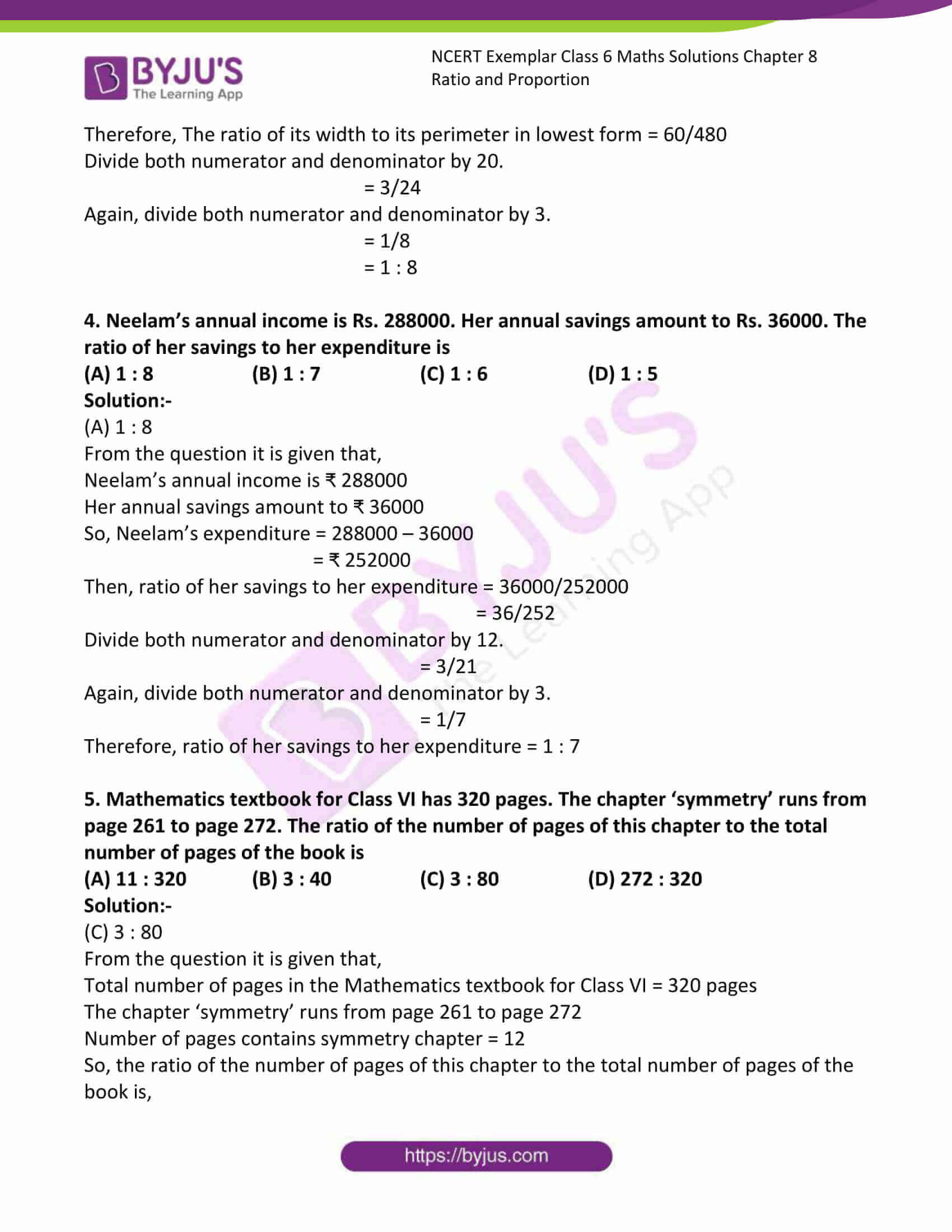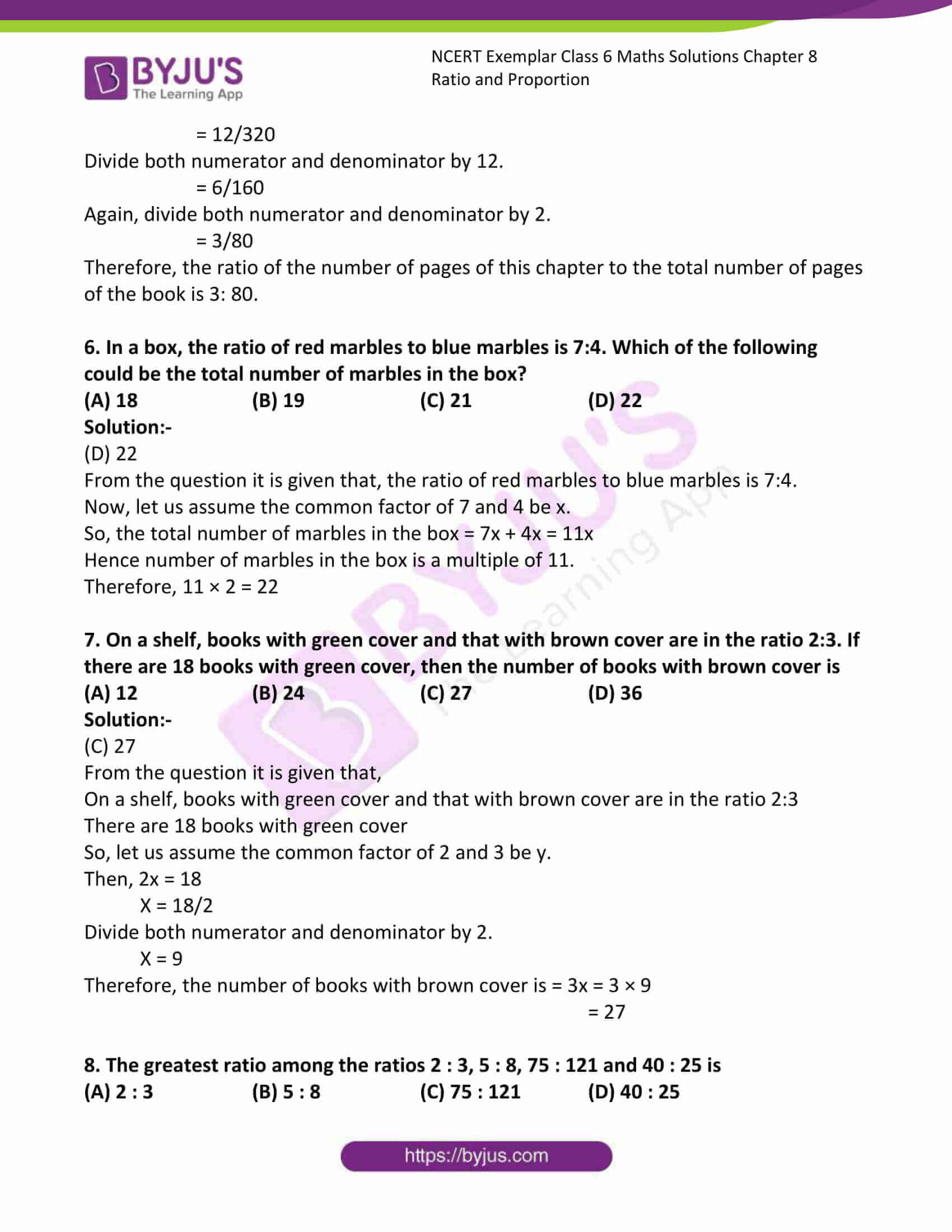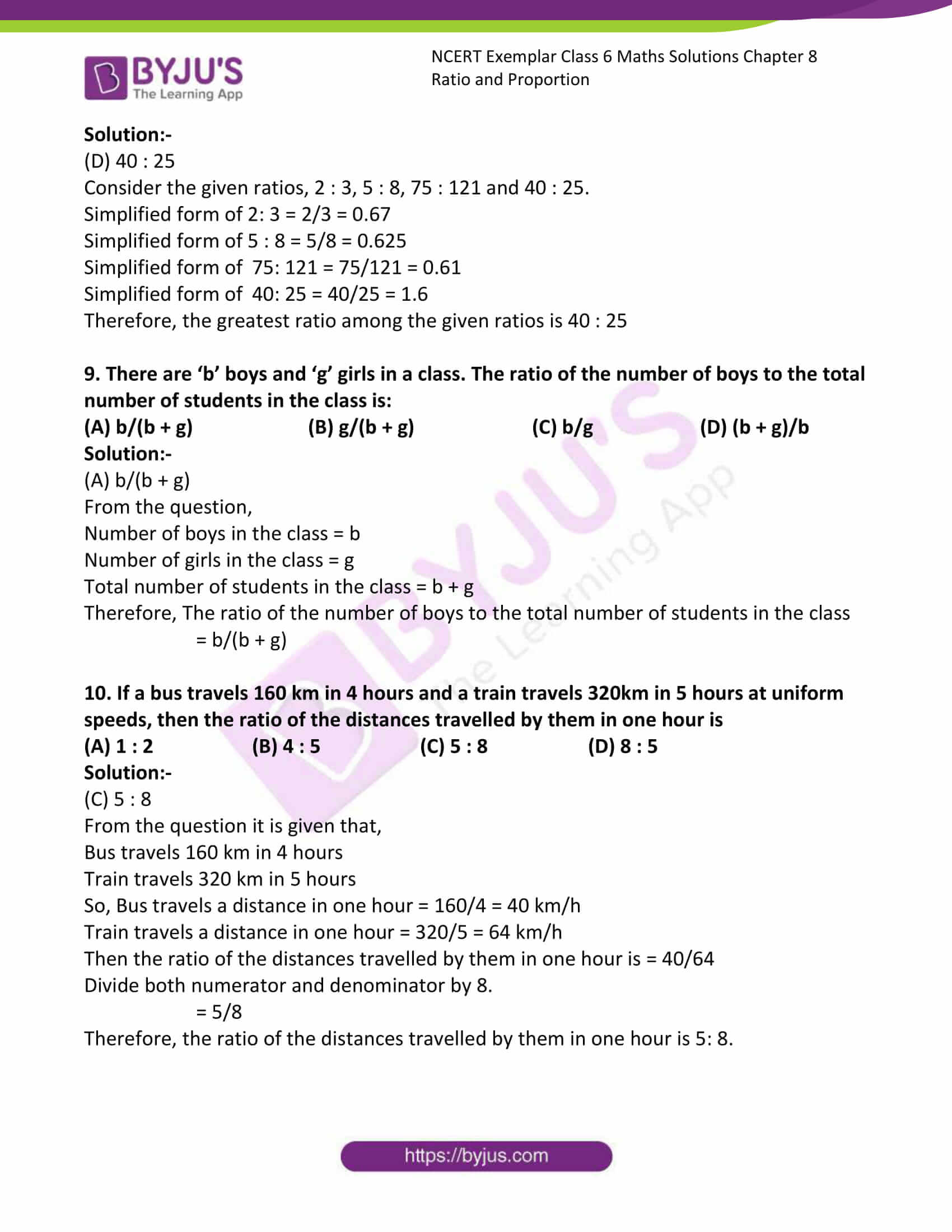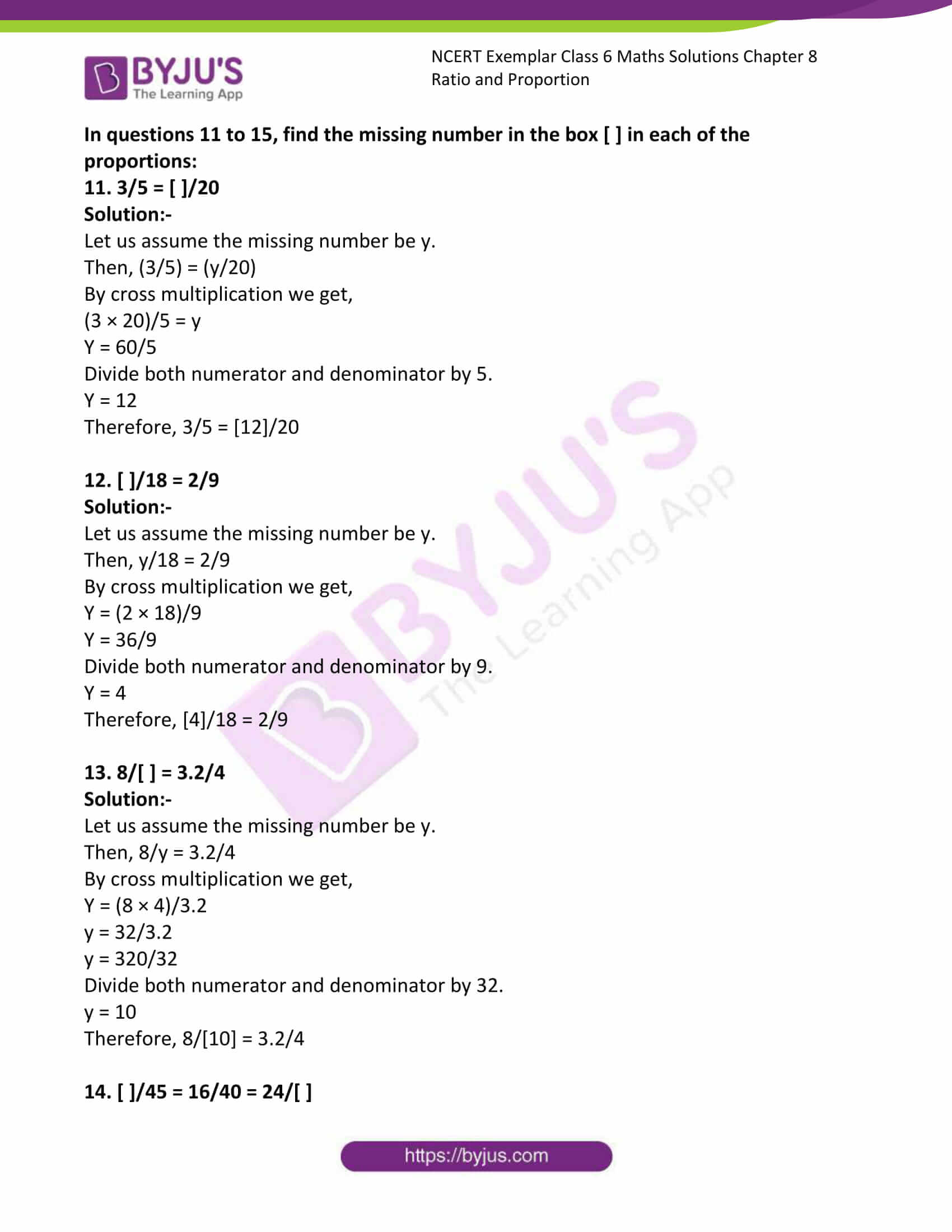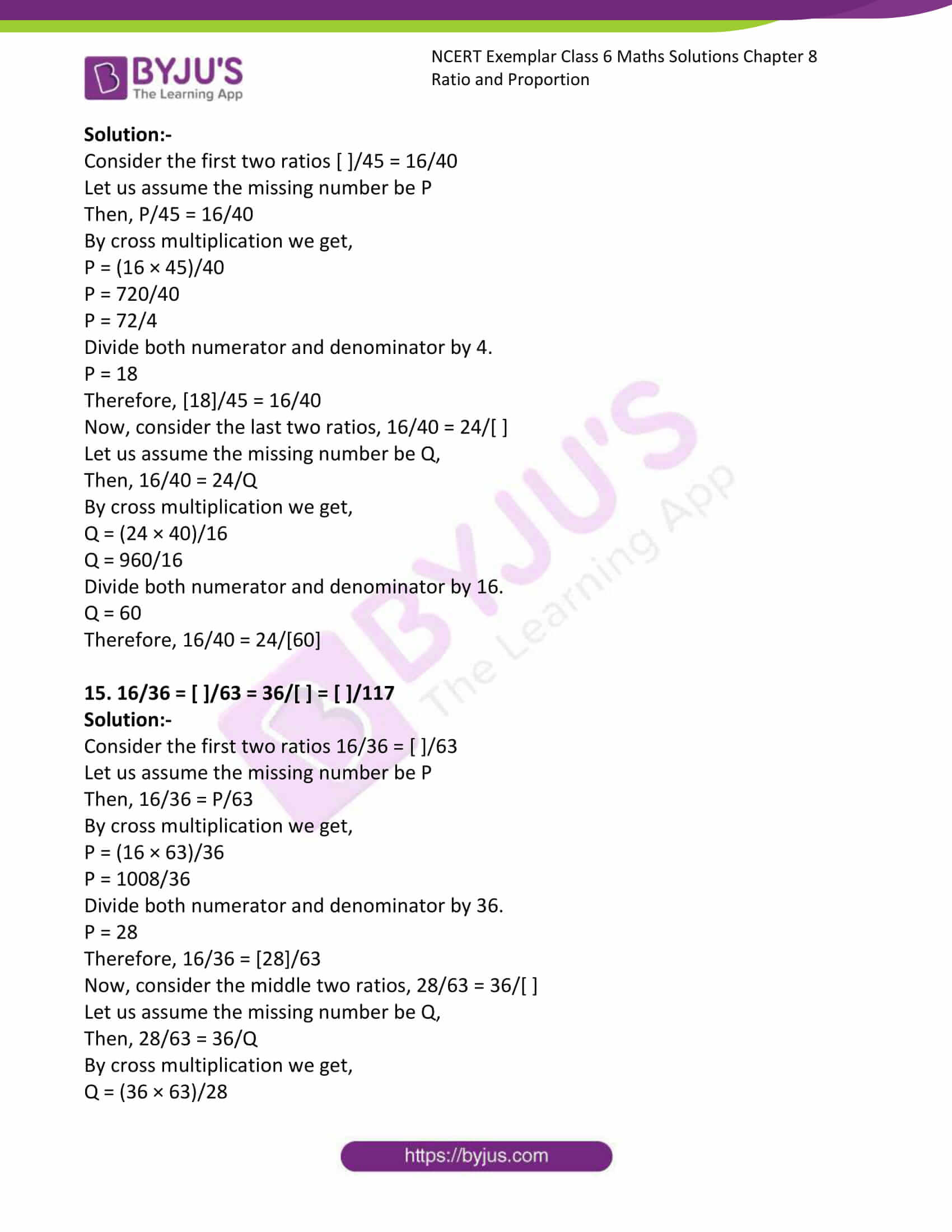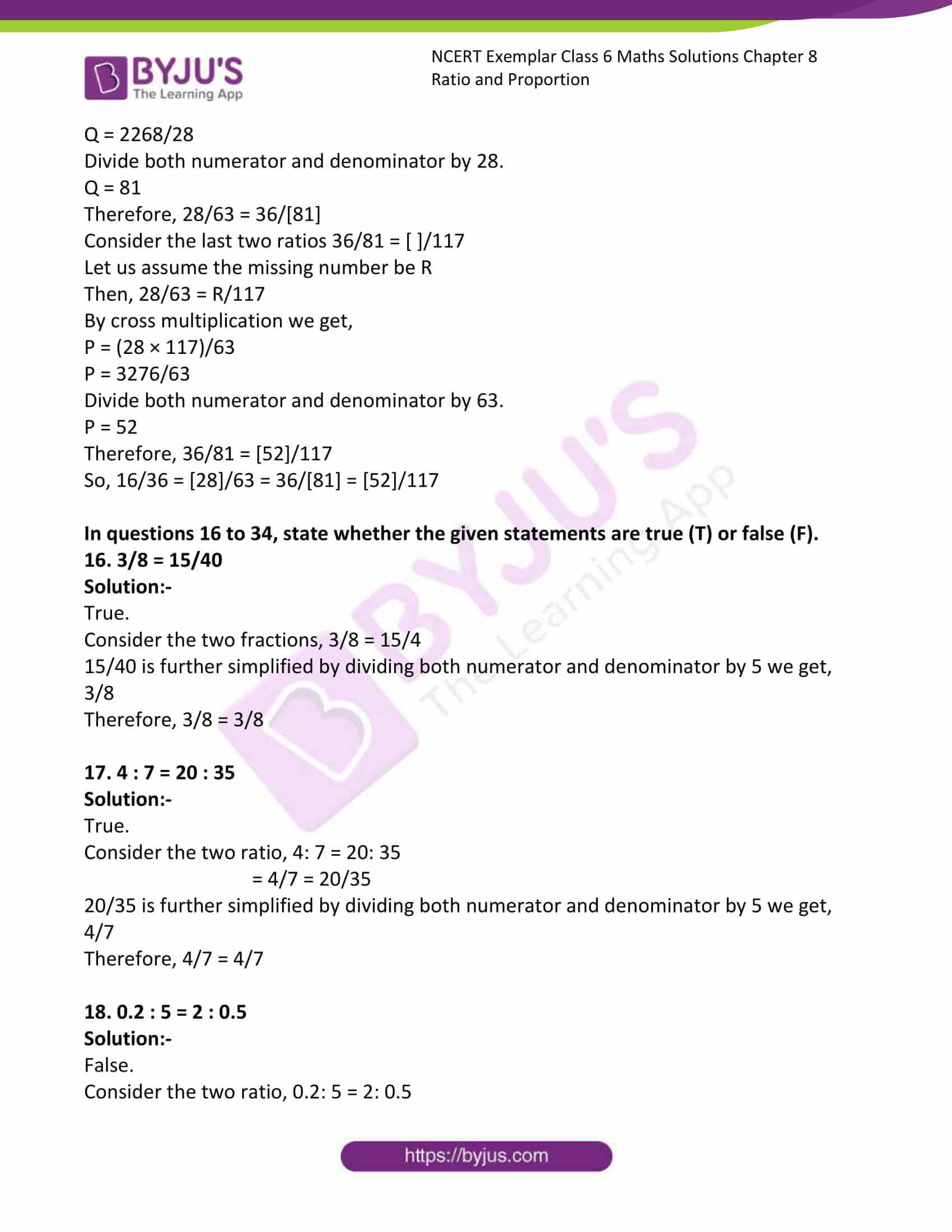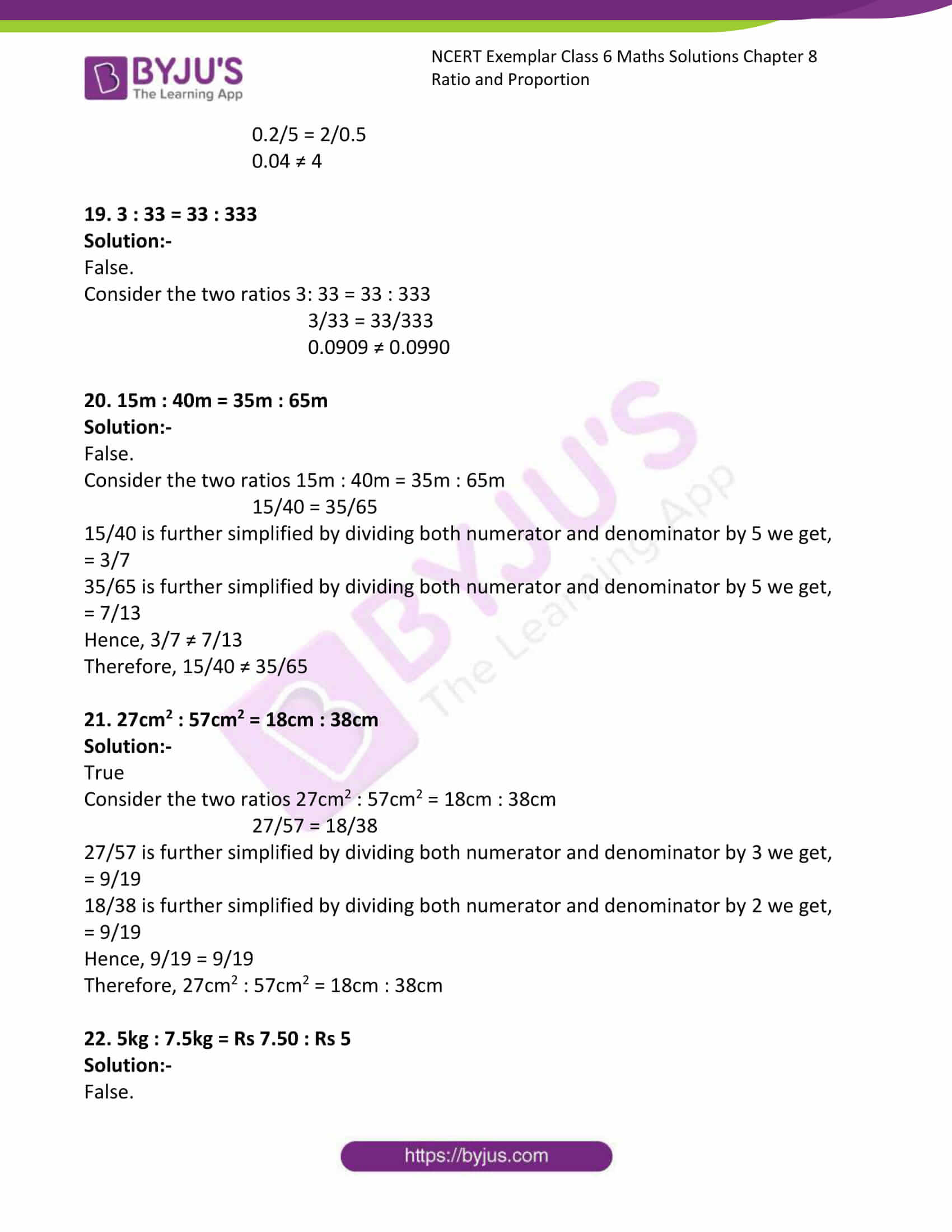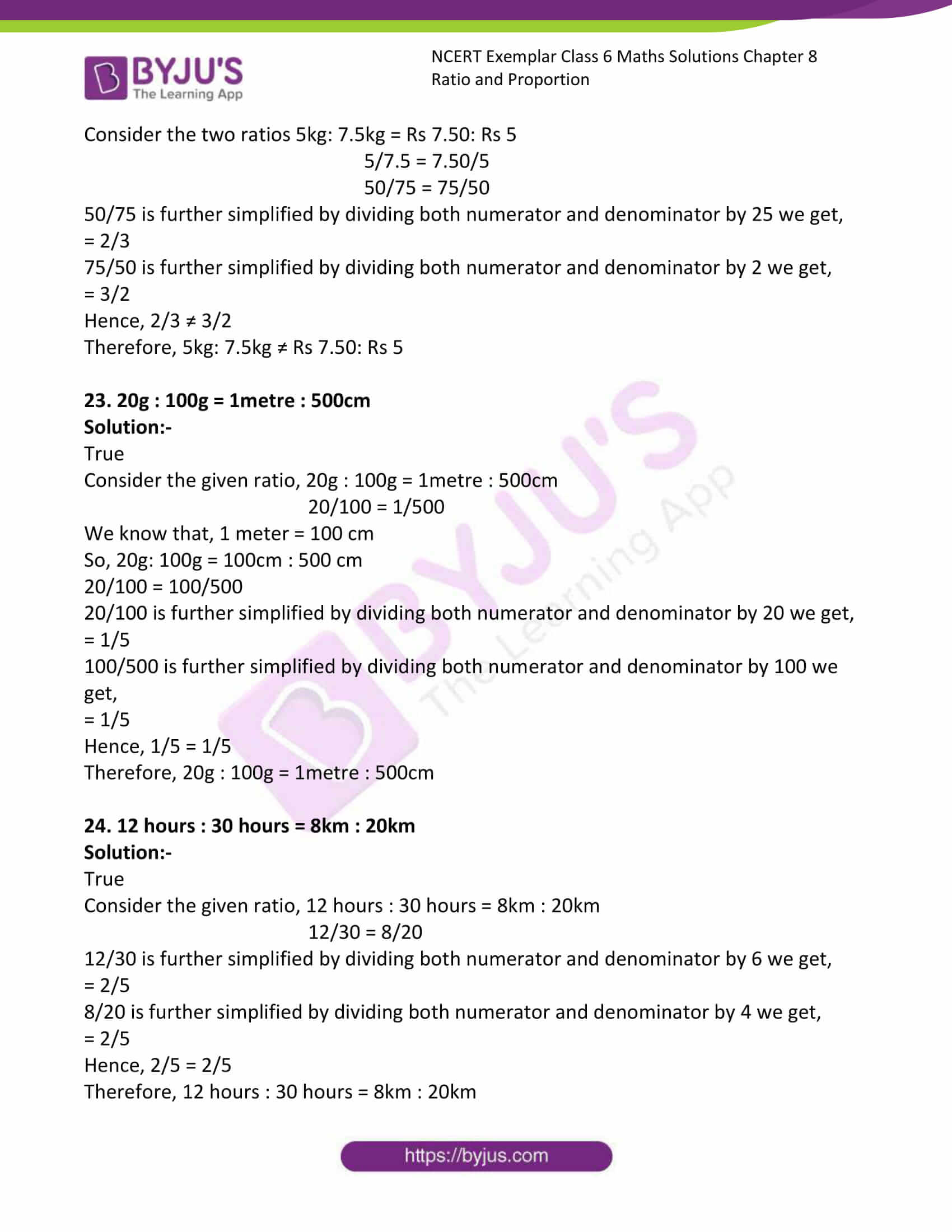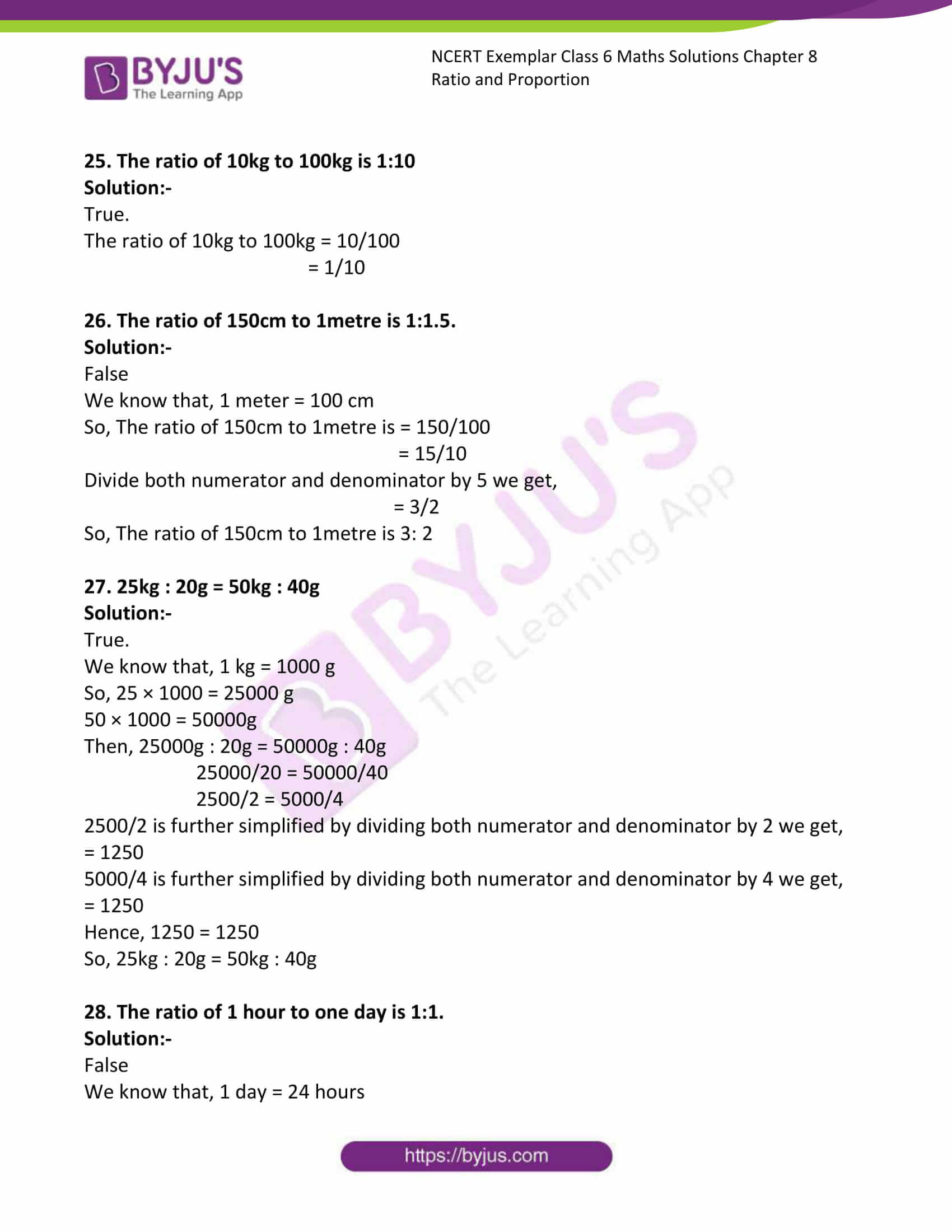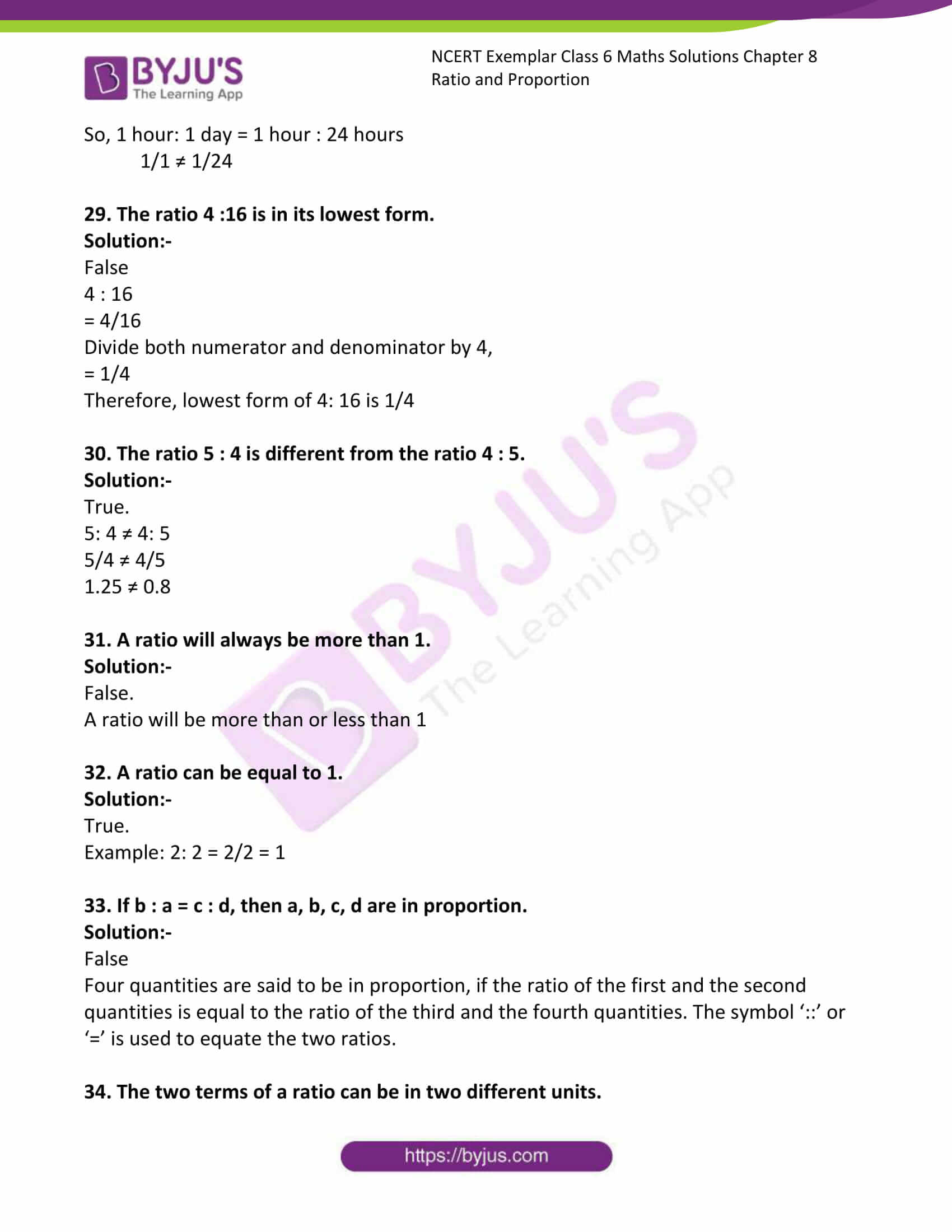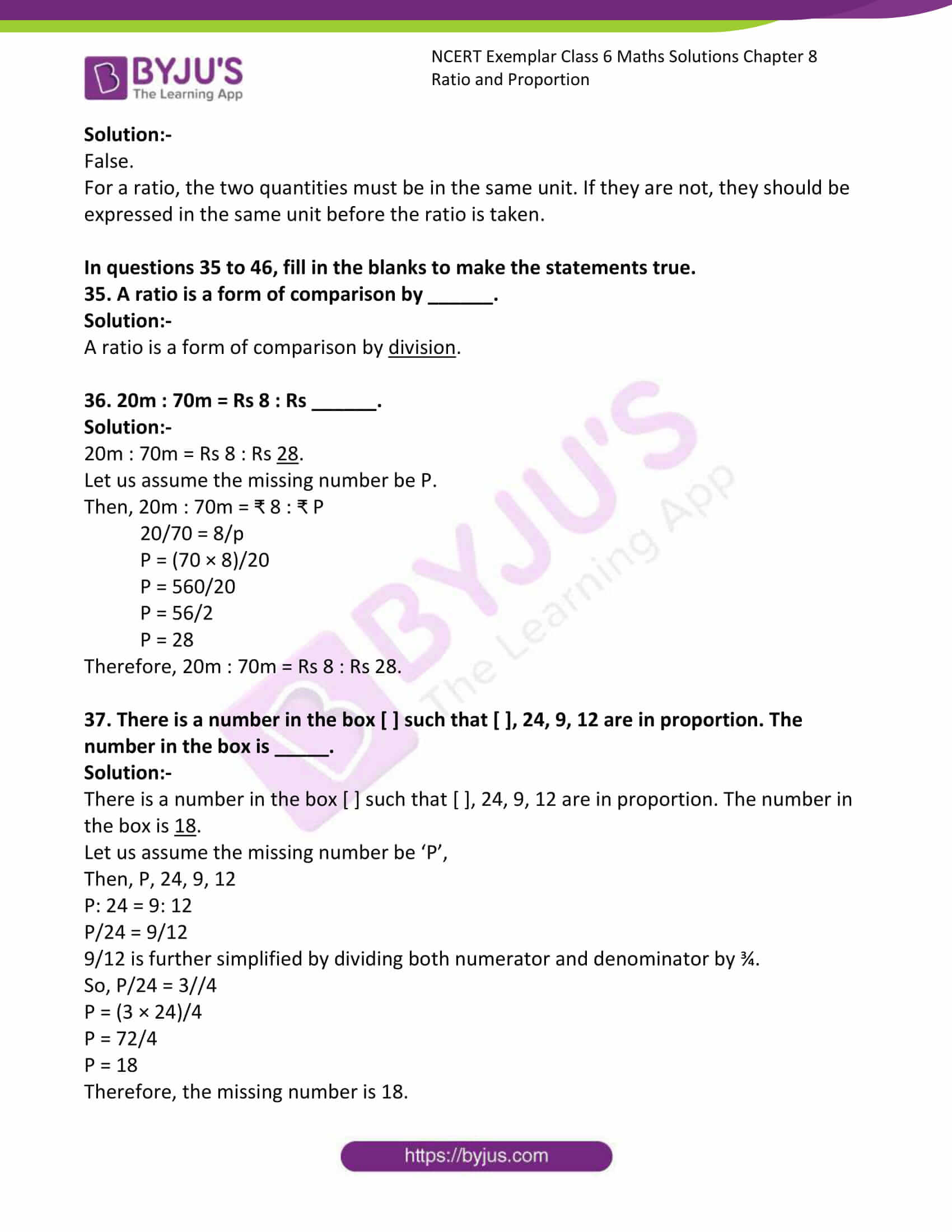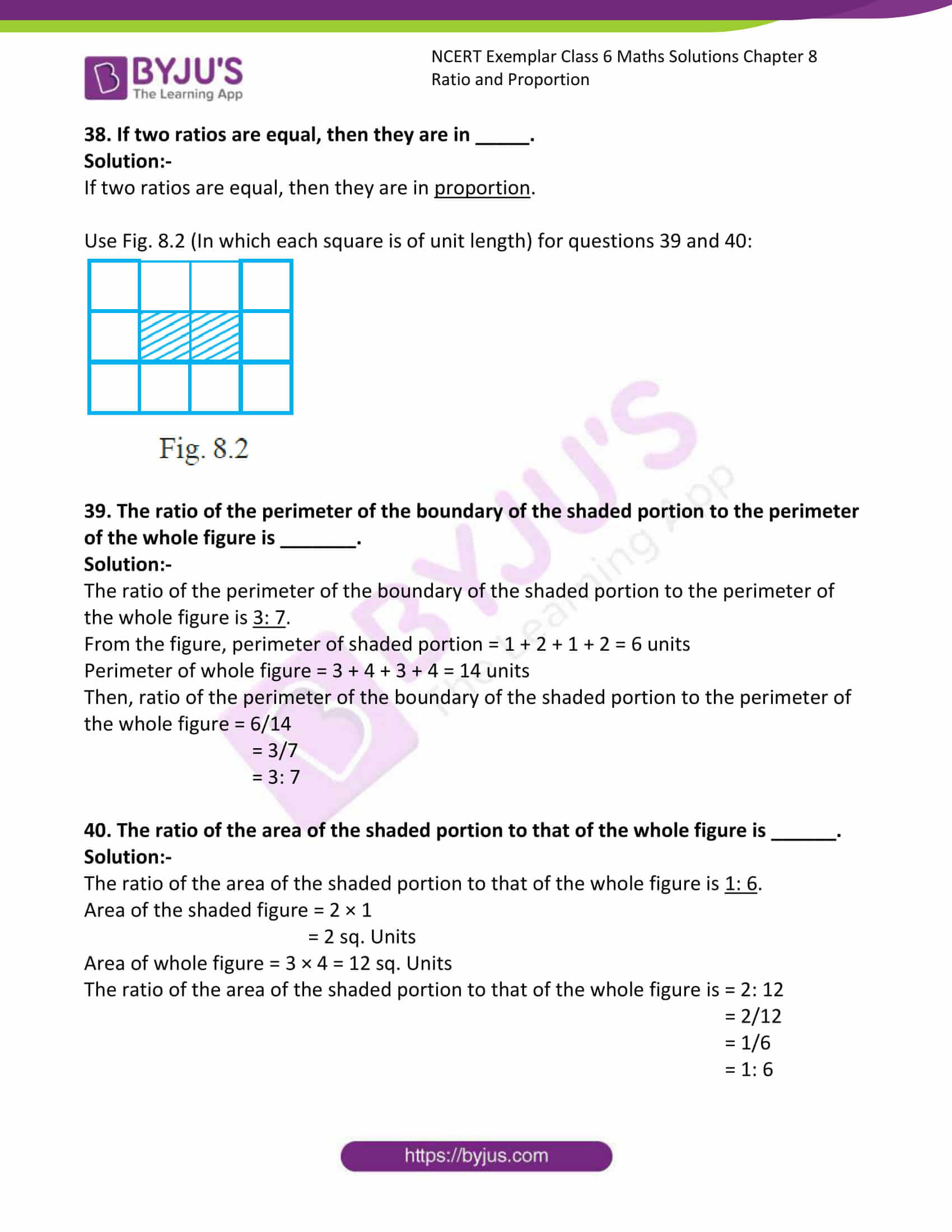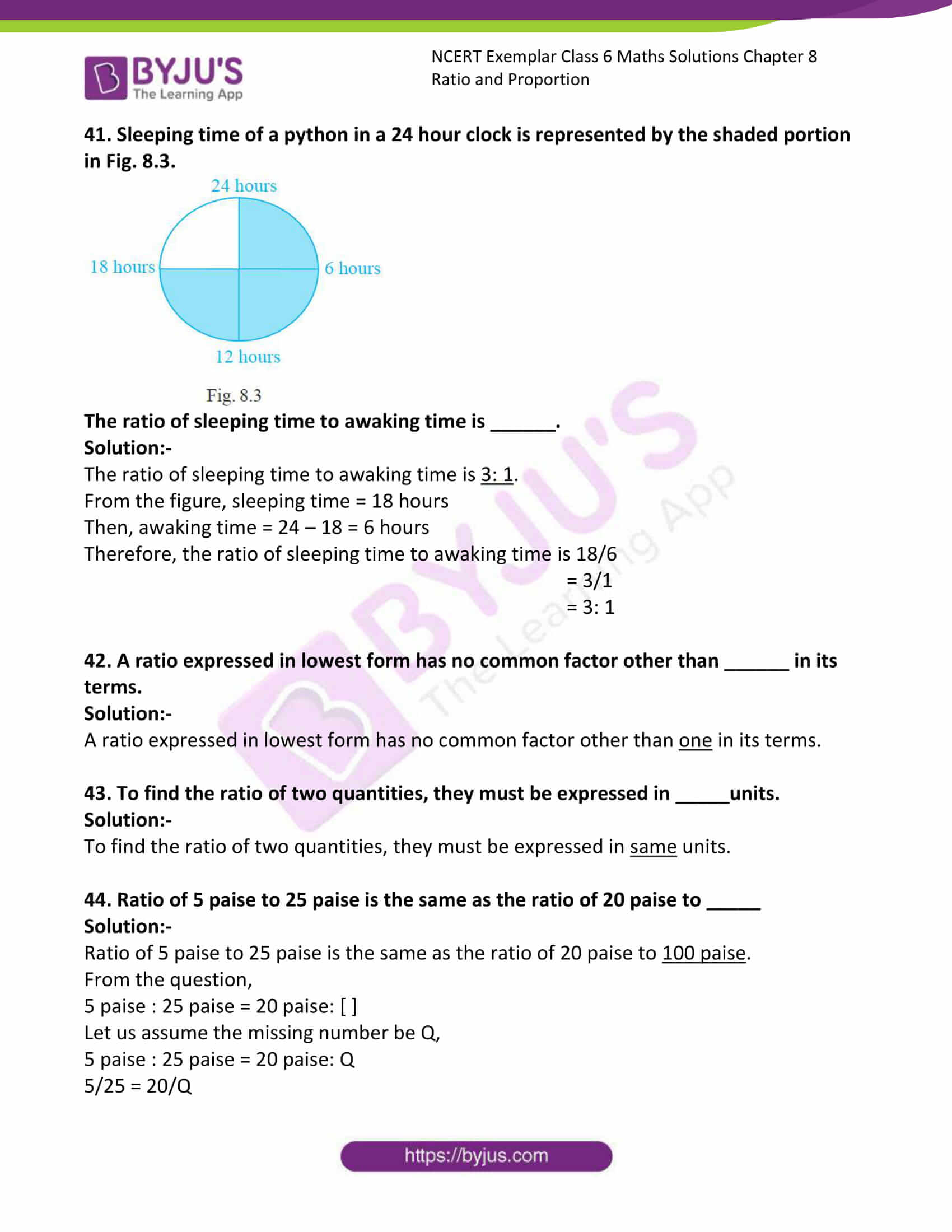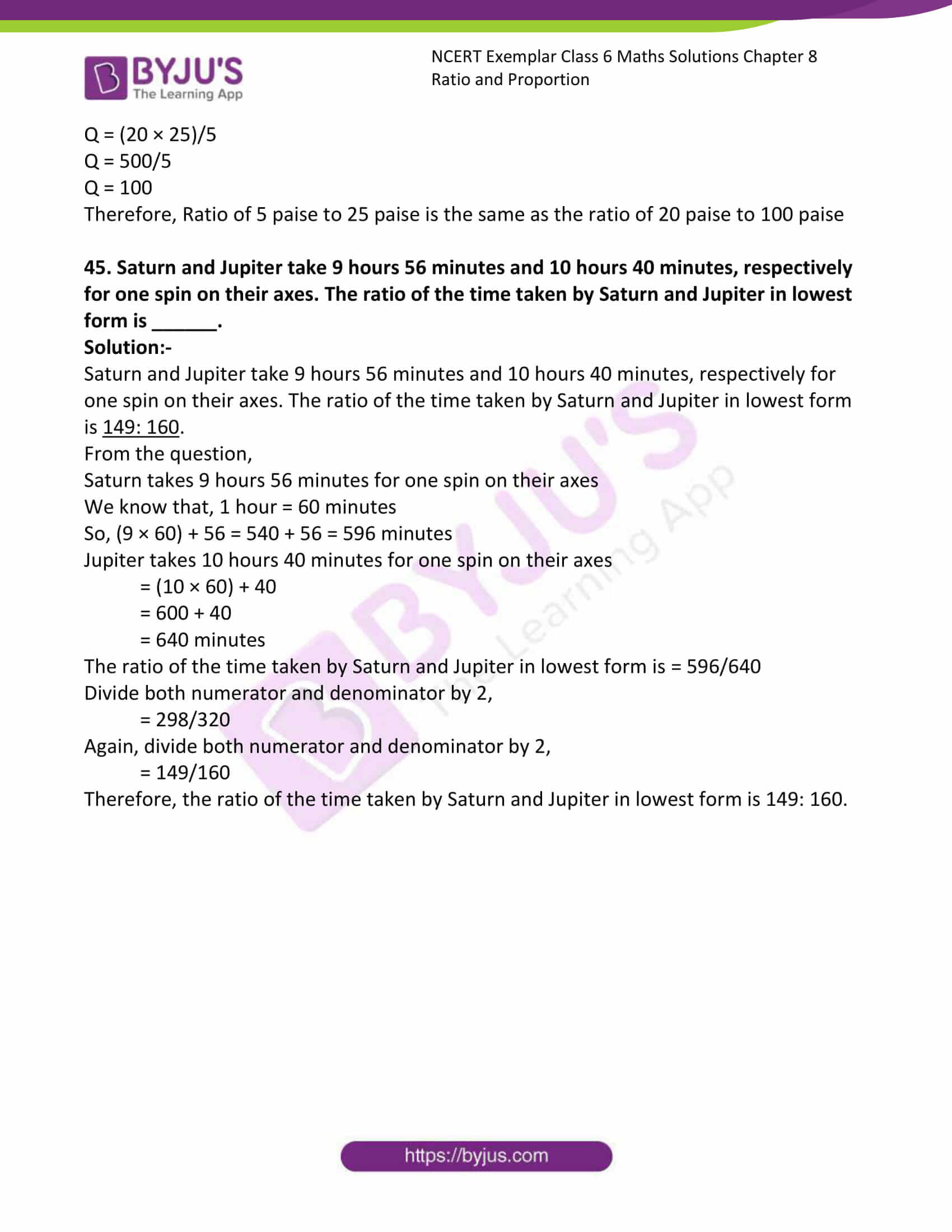### Access Answers to NCERT Exemplar Class 6 Maths Chapter 8 Ratio and Proportion

Exercise Page: 120

In questions 1 to 10, only one of the four options is correct. Write the correct one.

1. The ratio of 8 books to 20 books is

(A) 2 : 5 (B) 5 : 2 (C) 4 : 5 (D) 5 : 4

Solution:-

(A) 2 : 5

The comparison of two numbers or quantities by division is known as the ratio. Symbol ‘:’ is used to denote ratio.

Ratio of 8 books to 20 books = 8/20

Divide both numerator and denominator by 4.

= 2/5

Therefore, ratio of 8 books to 20 books = 2 : 5

2. The ratio of the number of sides of a square to the number of edges of a cube is

(A) 1 : 2 (B) 3 : 2 (C) 4 : 1 (D) 1 : 3

Solution:-

(D) 1 : 3

We know that, number of sides in a square = 4 and number of edges in a cube = 12

So, ratio of sides to edges = 4/12

Divide both numerator and denominator by 4.

= 1/3

Therefore, ratio of sides to edges = 1 : 3

3. A picture is 60cm wide and 1.8m long. The ratio of its width to its perimeter in lowest form is

(A) 1 : 2 (B) 1 : 3 (C) 1 : 4 (D) 1 : 8

Solution:-

(D) 1 : 8

From the question it is given that,

Width of a picture = 60 cm

Length of a picture = 1.8 m

We know that, 1 m = 100 cm

So, 1.8 m = 180 cm

Perimeter of rectangle = 2 (length + breadth)

= 2 (180 + 60)

= 2 (240)

= 480

Therefore, The ratio of its width to its perimeter in lowest form = 60/480

Divide both numerator and denominator by 20.

= 3/24

Again, divide both numerator and denominator by 3.

= 1/8

= 1 : 8

4. Neelam’s annual income is Rs. 288000. Her annual savings amount to Rs. 36000. The ratio of her savings to her expenditure is

(A) 1 : 8 (B) 1 : 7 (C) 1 : 6 (D) 1 : 5

Solution:-

(A) 1 : 8

From the question it is given that,

Neelam’s annual income is ₹ 288000

Her annual savings amount to ₹ 36000

So, Neelam’s expenditure = 288000 – 36000

= ₹ 252000

Then, ratio of her savings to her expenditure = 36000/252000

= 36/252

Divide both numerator and denominator by 12.

= 3/21

Again, divide both numerator and denominator by 3.

= 1/7

Therefore, ratio of her savings to her expenditure = 1 : 7

5. Mathematics textbook for Class VI has 320 pages. The chapter ‘symmetry’ runs from page 261 to page 272. The ratio of the number of pages of this chapter to the total number of pages of the book is

(A) 11 : 320 (B) 3 : 40 (C) 3 : 80 (D) 272 : 320

Solution:-

(C) 3 : 80

From the question it is given that,

Total number of pages in the Mathematics textbook for Class VI = 320 pages

The chapter ‘symmetry’ runs from page 261 to page 272

Number of pages contains symmetry chapter = 12

So, the ratio of the number of pages of this chapter to the total number of pages of the book is,

= 12/320

Divide both numerator and denominator by 12.

= 6/160

Again, divide both numerator and denominator by 2.

= 3/80

Therefore, the ratio of the number of pages of this chapter to the total number of pages of the book is 3: 80.

6. In a box, the ratio of red marbles to blue marbles is 7:4. Which of the following could be the total number of marbles in the box?

(A) 18 (B) 19 (C) 21 (D) 22

Solution:-

(D) 22

From the question it is given that, the ratio of red marbles to blue marbles is 7:4.

Now, let us assume the common factor of 7 and 4 be x.

So, the total number of marbles in the box = 7x + 4x = 11x

Hence number of marbles in the box is a multiple of 11.

Therefore, 11 × 2 = 22

7. On a shelf, books with green cover and that with brown cover are in the ratio 2:3. If there are 18 books with green cover, then the number of books with brown cover is

(A) 12 (B) 24 (C) 27 (D) 36

Solution:-

(C) 27

From the question it is given that,

On a shelf, books with green cover and that with brown cover are in the ratio 2:3

There are 18 books with green cover

So, let us assume the common factor of 2 and 3 be y.

Then, 2x = 18

X = 18/2

Divide both numerator and denominator by 2.

X = 9

Therefore, the number of books with brown cover is = 3x = 3 × 9

= 27

8. The greatest ratio among the ratios 2 : 3, 5 : 8, 75 : 121 and 40 : 25 is

(A) 2 : 3 (B) 5 : 8 (C) 75 : 121 (D) 40 : 25

Solution:-

(D) 40 : 25

Consider the given ratios, 2 : 3, 5 : 8, 75 : 121 and 40 : 25.

Simplified form of 2: 3 = 2/3 = 0.67

Simplified form of 5 : 8 = 5/8 = 0.625

Simplified form of 75: 121 = 75/121 = 0.61

Simplified form of 40: 25 = 40/25 = 1.6

Therefore, the greatest ratio among the given ratios is 40 : 25

9. There are ‘b’ boys and ‘g’ girls in a class. The ratio of the number of boys to the total number of students in the class is:

(A) b/(b + g) (B) g/(b + g) (C) b/g (D) (b + g)/b

Solution:-

(A) b/(b + g)

From the question,

Number of boys in the class = b

Number of girls in the class = g

Total number of students in the class = b + g

Therefore, The ratio of the number of boys to the total number of students in the class

= b/(b + g)

10. If a bus travels 160 km in 4 hours and a train travels 320km in 5 hours at uniform speeds, then the ratio of the distances travelled by them in one hour is

(A) 1 : 2 (B) 4 : 5 (C) 5 : 8 (D) 8 : 5

Solution:-

(C) 5 : 8

From the question it is given that,

Bus travels 160 km in 4 hours

Train travels 320 km in 5 hours

So, Bus travels a distance in one hour = 160/4 = 40 km/h

Train travels a distance in one hour = 320/5 = 64 km/h

Then the ratio of the distances travelled by them in one hour is = 40/64

Divide both numerator and denominator by 8.

= 5/8

Therefore, the ratio of the distances travelled by them in one hour is 5: 8.

In questions 11 to 15, find the missing number in the box [ ] in each of the proportions:

11. 3/5 = [ ]/20

Solution:-

Let us assume the missing number be y.

Then, (3/5) = (y/20)

By cross multiplication we get,

(3 × 20)/5 = y

Y = 60/5

Divide both numerator and denominator by 5.

Y = 12

Therefore, 3/5 = /20

12. [ ]/18 = 2/9

Solution:-

Let us assume the missing number be y.

Then, y/18 = 2/9

By cross multiplication we get,

Y = (2 × 18)/9

Y = 36/9

Divide both numerator and denominator by 9.

Y = 4

Therefore, /18 = 2/9

13. 8/[ ] = 3.2/4

Solution:-

Let us assume the missing number be y.

Then, 8/y = 3.2/4

By cross multiplication we get,

Y = (8 × 4)/3.2

y = 32/3.2

y = 320/32

Divide both numerator and denominator by 32.

y = 10

Therefore, 8/ = 3.2/4

14. [ ]/45 = 16/40 = 24/[ ]

Solution:-

Consider the first two ratios [ ]/45 = 16/40

Let us assume the missing number be P

Then, P/45 = 16/40

By cross multiplication we get,

P = (16 × 45)/40

P = 720/40

P = 72/4

Divide both numerator and denominator by 4.

P = 18

Therefore, /45 = 16/40

Now, consider the last two ratios, 16/40 = 24/[ ]

Let us assume the missing number be Q,

Then, 16/40 = 24/Q

By cross multiplication we get,

Q = (24 × 40)/16

Q = 960/16

Divide both numerator and denominator by 16.

Q = 60

Therefore, 16/40 = 24/

15. 16/36 = [ ]/63 = 36/[ ] = [ ]/117

Solution:-

Consider the first two ratios 16/36 = [ ]/63

Let us assume the missing number be P

Then, 16/36 = P/63

By cross multiplication we get,

P = (16 × 63)/36

P = 1008/36

Divide both numerator and denominator by 36.

P = 28

Therefore, 16/36 = /63

Now, consider the middle two ratios, 28/63 = 36/[ ]

Let us assume the missing number be Q,

Then, 28/63 = 36/Q

By cross multiplication we get,

Q = (36 × 63)/28

Q = 2268/28

Divide both numerator and denominator by 28.

Q = 81

Therefore, 28/63 = 36/

Consider the last two ratios 36/81 = [ ]/117

Let us assume the missing number be R

Then, 28/63 = R/117

By cross multiplication we get,

P = (28 × 117)/63

P = 3276/63

Divide both numerator and denominator by 63.

P = 52

Therefore, 36/81 = /117

So, 16/36 = /63 = 36/ = /117

In questions 16 to 34, state whether the given statements are true (T) or false (F).

16. 3/8 = 15/40

Solution:-

True.

Consider the two fractions, 3/8 = 15/4

15/40 is further simplified by dividing both numerator and denominator by 5 we get,

3/8

Therefore, 3/8 = 3/8

17. 4 : 7 = 20 : 35

Solution:-

True.

Consider the two ratio, 4: 7 = 20: 35

= 4/7 = 20/35

20/35 is further simplified by dividing both numerator and denominator by 5 we get,

4/7

Therefore, 4/7 = 4/7

18. 0.2 : 5 = 2 : 0.5

Solution:-

False.

Consider the two ratio, 0.2: 5 = 2: 0.5

0.2/5 = 2/0.5

0.04 ≠ 4

19. 3 : 33 = 33 : 333

Solution:-

False.

Consider the two ratios 3: 33 = 33 : 333

3/33 = 33/333

0.0909 ≠ 0.0990

20. 15m : 40m = 35m : 65m

Solution:-

False.

Consider the two ratios 15m : 40m = 35m : 65m

15/40 = 35/65

15/40 is further simplified by dividing both numerator and denominator by 5 we get,

= 3/7

35/65 is further simplified by dividing both numerator and denominator by 5 we get,

= 7/13

Hence, 3/7 ≠ 7/13

Therefore, 15/40 ≠ 35/65

21. 27cm2 : 57cm2 = 18cm : 38cm

Solution:-

True

Consider the two ratios 27cm2 : 57cm2 = 18cm : 38cm

27/57 = 18/38

27/57 is further simplified by dividing both numerator and denominator by 3 we get,

= 9/19

18/38 is further simplified by dividing both numerator and denominator by 2 we get,

= 9/19

Hence, 9/19 = 9/19

Therefore, 27cm2 : 57cm2 = 18cm : 38cm

22. 5kg : 7.5kg = Rs 7.50 : Rs 5

Solution:-

False.

Consider the two ratios 5kg: 7.5kg = Rs 7.50: Rs 5

5/7.5 = 7.50/5

50/75 = 75/50

50/75 is further simplified by dividing both numerator and denominator by 25 we get,

= 2/3

75/50 is further simplified by dividing both numerator and denominator by 2 we get,

= 3/2

Hence, 2/3 ≠ 3/2

Therefore, 5kg: 7.5kg ≠ Rs 7.50: Rs 5

23. 20g : 100g = 1metre : 500cm

Solution:-

True

Consider the given ratio, 20g : 100g = 1metre : 500cm

20/100 = 1/500

We know that, 1 meter = 100 cm

So, 20g: 100g = 100cm : 500 cm

20/100 = 100/500

20/100 is further simplified by dividing both numerator and denominator by 20 we get,

= 1/5

100/500 is further simplified by dividing both numerator and denominator by 100 we get,

= 1/5

Hence, 1/5 = 1/5

Therefore, 20g : 100g = 1metre : 500cm

24. 12 hours : 30 hours = 8km : 20km

Solution:-

True

Consider the given ratio, 12 hours : 30 hours = 8km : 20km

12/30 = 8/20

12/30 is further simplified by dividing both numerator and denominator by 6 we get,

= 2/5

8/20 is further simplified by dividing both numerator and denominator by 4 we get,

= 2/5

Hence, 2/5 = 2/5

Therefore, 12 hours : 30 hours = 8km : 20km

25. The ratio of 10kg to 100kg is 1:10

Solution:-

True.

The ratio of 10kg to 100kg = 10/100

= 1/10

26. The ratio of 150cm to 1metre is 1:1.5.

Solution:-

False

We know that, 1 meter = 100 cm

So, The ratio of 150cm to 1metre is = 150/100

= 15/10

Divide both numerator and denominator by 5 we get,

= 3/2

So, The ratio of 150cm to 1metre is 3: 2

27. 25kg : 20g = 50kg : 40g

Solution:-

True.

We know that, 1 kg = 1000 g

So, 25 × 1000 = 25000 g

50 × 1000 = 50000g

Then, 25000g : 20g = 50000g : 40g

25000/20 = 50000/40

2500/2 = 5000/4

2500/2 is further simplified by dividing both numerator and denominator by 2 we get,

= 1250

5000/4 is further simplified by dividing both numerator and denominator by 4 we get,

= 1250

Hence, 1250 = 1250

So, 25kg : 20g = 50kg : 40g

28. The ratio of 1 hour to one day is 1:1.

Solution:-

False

We know that, 1 day = 24 hours

So, 1 hour: 1 day = 1 hour : 24 hours

1/1 ≠ 1/24

29. The ratio 4 :16 is in its lowest form.

Solution:-

False

4 : 16

= 4/16

Divide both numerator and denominator by 4,

= 1/4

Therefore, lowest form of 4: 16 is 1/4

30. The ratio 5 : 4 is different from the ratio 4 : 5.

Solution:-

True.

5: 4 ≠ 4: 5

5/4 ≠ 4/5

1.25 ≠ 0.8

31. A ratio will always be more than 1.

Solution:-

False.

A ratio will be more than or less than 1

32. A ratio can be equal to 1.

Solution:-

True.

Example: 2: 2 = 2/2 = 1

33. If b : a = c : d, then a, b, c, d are in proportion.

Solution:-

False

Four quantities are said to be in proportion, if the ratio of the first and the second quantities is equal to the ratio of the third and the fourth quantities. The symbol ‘::’ or ‘=’ is used to equate the two ratios.

34. The two terms of a ratio can be in two different units.

Solution:-

False.

For a ratio, the two quantities must be in the same unit. If they are not, they should be expressed in the same unit before the ratio is taken.

In questions 35 to 46, fill in the blanks to make the statements true.

35. A ratio is a form of comparison by ______.

Solution:-

A ratio is a form of comparison by division.

36. 20m : 70m = Rs 8 : Rs ______.

Solution:-

20m : 70m = Rs 8 : Rs 28.

Let us assume the missing number be P.

Then, 20m : 70m = ₹ 8 : ₹ P

20/70 = 8/p

P = (70 × 8)/20

P = 560/20

P = 56/2

P = 28

Therefore, 20m : 70m = Rs 8 : Rs 28.

37. There is a number in the box [ ] such that [ ], 24, 9, 12 are in proportion. The number in the box is _____.

Solution:-

There is a number in the box [ ] such that [ ], 24, 9, 12 are in proportion. The number in the box is 18.

Let us assume the missing number be ‘P’,

Then, P, 24, 9, 12

P: 24 = 9: 12

P/24 = 9/12

9/12 is further simplified by dividing both numerator and denominator by ¾.

So, P/24 = 3//4

P = (3 × 24)/4

P = 72/4

P = 18

Therefore, the missing number is 18.

38. If two ratios are equal, then they are in _____.

Solution:-

If two ratios are equal, then they are in proportion.

Use Fig. 8.2 (In which each square is of unit length) for questions 39 and 40: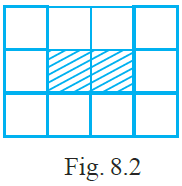39. The ratio of the perimeter of the boundary of the shaded portion to the perimeter of the whole figure is _______.

Solution:-

The ratio of the perimeter of the boundary of the shaded portion to the perimeter of the whole figure is 3: 7.

From the figure, perimeter of shaded portion = 1 + 2 + 1 + 2 = 6 units

Perimeter of whole figure = 3 + 4 + 3 + 4 = 14 units

Then, ratio of the perimeter of the boundary of the shaded portion to the perimeter of the whole figure = 6/14

= 3/7

= 3: 7

40. The ratio of the area of the shaded portion to that of the whole figure is ______.

Solution:-

The ratio of the area of the shaded portion to that of the whole figure is 1: 6.

Area of the shaded figure = 2 × 1

= 2 sq. Units

Area of whole figure = 3 × 4 = 12 sq. Units

The ratio of the area of the shaded portion to that of the whole figure is = 2: 12

= 2/12

= 1/6

= 1: 6

41. Sleeping time of a python in a 24 hour clock is represented by the shaded portion in Fig. 8.3.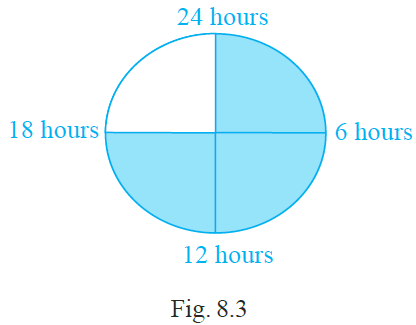The ratio of sleeping time to awaking time is ______.

Solution:-

The ratio of sleeping time to awaking time is 3: 1.

From the figure, sleeping time = 18 hours

Then, awaking time = 24 – 18 = 6 hours

Therefore, the ratio of sleeping time to awaking time is 18/6

= 3/1

= 3: 1

42. A ratio expressed in lowest form has no common factor other than ______ in its terms.

Solution:-

A ratio expressed in lowest form has no common factor other than one in its terms.

43. To find the ratio of two quantities, they must be expressed in _____units.

Solution:-

To find the ratio of two quantities, they must be expressed in same units.

44. Ratio of 5 paise to 25 paise is the same as the ratio of 20 paise to _____

Solution:-

Ratio of 5 paise to 25 paise is the same as the ratio of 20 paise to 100 paise.

From the question,

5 paise : 25 paise = 20 paise: [ ]

Let us assume the missing number be Q,

5 paise : 25 paise = 20 paise: Q

5/25 = 20/Q

Q = (20 × 25)/5

Q = 500/5

Q = 100

Therefore, Ratio of 5 paise to 25 paise is the same as the ratio of 20 paise to 100 paise

45. Saturn and Jupiter take 9 hours 56 minutes and 10 hours 40 minutes, respectively for one spin on their axes. The ratio of the time taken by Saturn and Jupiter in lowest form is ______.

Solution:-

Saturn and Jupiter take 9 hours 56 minutes and 10 hours 40 minutes, respectively for one spin on their axes. The ratio of the time taken by Saturn and Jupiter in lowest form is 149: 160.

From the question,

Saturn takes 9 hours 56 minutes for one spin on their axes

We know that, 1 hour = 60 minutes

So, (9 × 60) + 56 = 540 + 56 = 596 minutes

Jupiter takes 10 hours 40 minutes for one spin on their axes

= (10 × 60) + 40

= 600 + 40

= 640 minutes

The ratio of the time taken by Saturn and Jupiter in lowest form is = 596/640

Divide both numerator and denominator by 2,

= 298/320

Again, divide both numerator and denominator by 2,

= 149/160

Therefore, the ratio of the time taken by Saturn and Jupiter in lowest form is 149: 160.